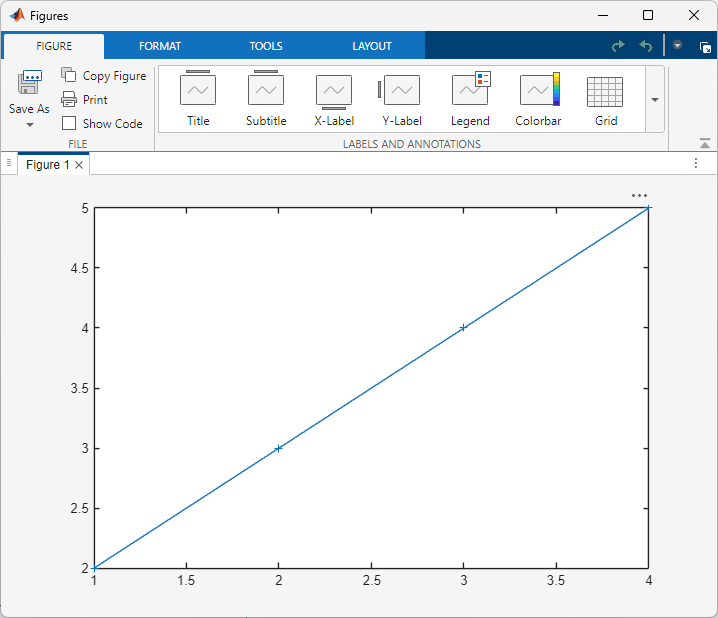## Debug MATLAB Function Blocks

You can use the MATLAB Function Block Editor to debug MATLAB Function block code. The MATLAB Function Block Editor includes some of the same debugging tools available in the MATLAB® editor. To debug MATLAB Function block code, set at least one breakpoint and run the simulation.

### Debug an Example Function

This example uses the model `call_stats_block2`, which you create in Create Custom Functionality Using MATLAB Function Blocks. To debug the MATLAB Function block code in this model:

1. Open the `call_stats_block2` model. Double-click the MATLAB Function block to open the editor.

2. In the MATLAB Function Block Editor, click the line number to the left of the line:

`len = length(vals);`

The line number is highlighted in red, which indicates the breakpoint.3. Simulate the model.

The simulation pauses when execution reaches the breakpoint. A green arrow points to where the simulation has paused.4. On the Function tab, click Step Over to advance execution.

The execution advances to the next line of `stats`, which calls the local function `avg`.

5. On the Function tab, click Step In.

Execution advances to the local function `avg`. Once you are in a local function, you can use the Step Over or Step In commands to advance execution. If the local function calls another local function, use Step In to enter it. If you want to execute the remaining lines of the local function, use Step Out.

6. Click Step Over to execute the only line in `avg`.

7. Click Step Over to return to the function `stats`.

Execution advances to the line after the call to `avg`.

8. Click Step Over twice to calculate the `stdev` and to execute the `plot` function.

The `plot` function executes in MATLAB:9. Click Continue to continue executing the model.

If you return to the model, the computed values of `mean` and `stdev` appear in the Display blocks.

10. In the MATLAB Function Block Editor, click to stop the simulation.

Note

You can debug MATLAB Function blocks in Normal and Accelerator modes. However, if you simulate a model in Accelerator mode that uses a MATLAB Function block in a model reference, the parent model simulation ignores the breakpoints.

### Set Conditions on Breakpoints

You can enter a MATLAB expression as a condition on a breakpoint inside a MATLAB Function block. Simulation then pauses on that breakpoint only when the condition is true. To set a conditional breakpoint, in the MATLAB Function Block Editor, right-click the line number to the left of a line of code and select Set Conditional Breakpoint, and enter the condition in the dialog box. You can use any valid MATLAB expression as a condition. This expression can include numerical values and any data that is in scope at the breakpoint.

To add or modify a condition on an existing breakpoint, right-click the breakpoint and select Set/Modify Condition.

### Watch Function Variables During Simulation

You can use several tools to track the variable values of the function while you simulate a MATLAB Function block.

#### Watch Function Variables with the Interactive Display

To display the value of a variable in the function of a MATLAB Function block during simulation:

1. Add a breakpoint to the MATLAB Function Block Editor. Run the model.

2. When the simulation pauses, point to a variable name. A tooltip displays the current variable value.

For example, to watch the variable `len` during simulation, point to `len` in the code.#### Watching Function Variables with the Command Line Debugger

You can view the values for a function variable in the Command Window during simulation. When you reach a breakpoint, enter the name of a variable at the `debug>> prompt` to see its value.

```debug>> stdev 1.1180 debug>> ```

You can also enter these commands in the command window while debugging:

CommandDescription

`dbcont`

Continue execution to next breakpoint.

`dbquit`

Quit debugging and terminate the simulation.

`dbstep [in|out]`

Advance to next program step after a breakpoint is encountered. Step over or step into/out of a MATLAB local function.

`help`

Display help for command line debugging.

`print <var>`

Display the value of the variable `var` in the current scope. If `var` is a vector or matrix, you can also index into `var`. For example, `var(1,2)`.

`save`

Saves all variables in the current scope to the specified file. Follows the syntax of the MATLAB `save` command. To retrieve variables from the MATLAB base workspace, use `load` command after simulation completes.

`<var>`

Equivalent to `print <var>` if the variable is in the current scope.

`who`

Display the variables in the current scope.

`whos`

Display the size and class (type) of all variables in the current scope.

If you enter other commands at the `debug>>` prompt, the results execute in the workspace of the MATLAB Function block. To issue a command in the MATLAB base workspace, use the `evalin` command with the first argument `'base'` followed by the second argument command, for example, `evalin('base','whos')`. To return to the MATLAB base workspace, use the `dbquit` command.

#### Display Size Limits

The MATLAB Function Block Editor does not display the contents of matrices that have more than two dimensions or more than `200` elements. For matrices that exceed these limits, the MATLAB Function Block Editor displays the shape and base type only.

### Check for Data Range Violations

MATLAB Function blocks check inputs and outputs for data range violations when the input or output values enter or leave the blocks. To enable data range violation checking, open the Configuration Parameters window, click Diagnostics > Data Validity, and set Simulation range checking to `error` or `warning`.

#### Specify a Data Range

To specify a range for input and output data, open the MATLAB Function Block Editor, click Ports & Data Manager, and select the input or output of interest. In the General tab, adjust the Limit range property by entering the desired values for Minimum and Maximum.